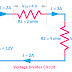Hi, in this article we are going to know about the voltage division. Also, we will know the examples of voltage dividers and the circuit diagram of voltage dividers.

## What is Voltage Division?

When a voltage is applied across an electrical or electronic circuit having multiple components connected in series, then the voltage drop across those components will be proportional to their resistance and the sum of all the voltage drop will be equal to the applied voltage. This is known as Voltage Division or Voltage Divider Rule. The voltage divider is also known as a potential divider. So, the voltage drop will be maximum across the resistor which having maximum resistance, and the voltage drop will be minimum across the resistor which has minimum resistance.

The voltage division rule is applicable for both AC and DC circuits. But for AC circuits, impedance(the algebraic sum of inductive reactance and capacitive reactance) also be considered when calculating the voltage drops. Also, remember that voltage drop not only happens in a pure series circuit, also happens in combinational circuits also. Calculation of voltage division is much easier for a pure series circuit than a combinational circuit.

You can see the effect of voltage division more if the circuit containing pure resistors and is connected to the DC supply. For AC circuits voltage drop depends upon the frequency of the supply. So, when calculating the voltage division for AC circuits, frequency, voltage, current all should be considered.

## Voltage Divider Circuit

Here, you can see a simple circuit diagram of a voltage divider having two series resistors and a DC power supply.

## Explanation of Voltage Divider Rule

Let's take the above circuit as an example to understand the voltage dividing rule. Here, you can see, the circuit is connected across a 12V power supply and having two resistors R1 = 2 ohms, R2 = 4 ohm.

The total resistance of the circuit is, (R1+R2) = (2+4) = 6 ohm

The current flow in the circuit is 12/6 = 2A

As per the voltage divider rule, the voltage drop will be,
Across Resistor(VR1) = 2 x 2 = 4V
Across Resistor(VR2) = 2 x 4 = 8V

Also, the algebraic sum of all voltage drop will be equal to the supply voltage.

Vs = VR1 + VR2
12 = 4+8
12=12

## Voltage Divider Formula

When you don't know the flow of current, then also you can calculate the voltage drop across each resistor using this formula.

VR1 = V[ R/ (R1+ R2+ R3+……+ Rn)]

VR2 = V[ R/ (R1+ R2+ R3+……+ Rn)]

......................................................................

......................................................................

VRn = V[ R/ (R1+ R2+ R3+……+ Rn)]

So, for the above circuit,

VR1 = V[R1/(R1+R2)]
= 12 x [2/(2+4)]
= 4V

VR2 = V[R2/(R1+R2)]
= 12 x [4/(2+4)]
= 8V

## Voltage Divider Examples and Applications

Potentiometer, volume control, series-connected lamps are the common examples of voltage dividers.

1. Voltage divider is used for voltage step-down purposes.

2. Voltage divider is used for motor speed control such as electrical fan regulator.

3. Voltage Dividers are used in LED Chaser Circuits.

4. Voltage divider circuit is used to produce reference voltage for analog circuits, comparators, etc.

5. Voltage divider is used in amplifier circuits, oscillator circuits, voltage regulator circuits, etc.

What is Voltage Division? Voltage Divider Example, CircuitReviewed by Author on 9/08/2021 Rating: 5